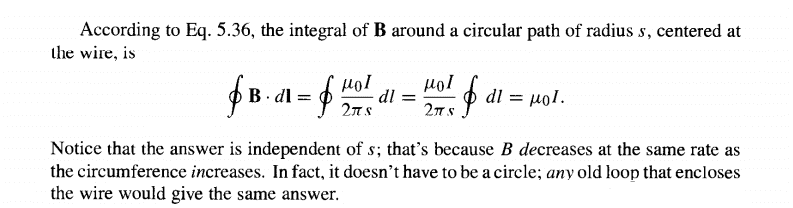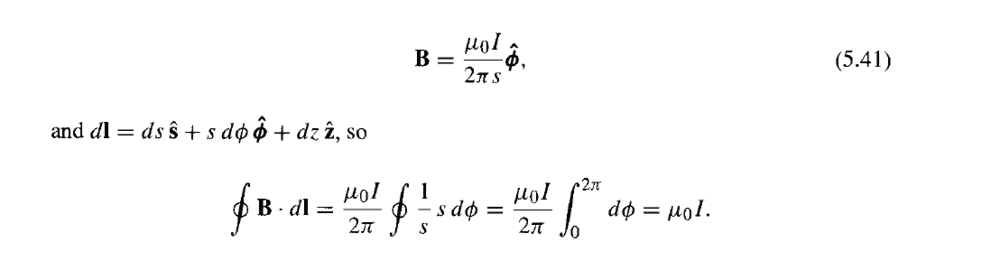# Why the magnetic field doesn't have to describe a circle?

Tags:
1. Mar 14, 2019

### JD_PM1. The problem statement, all variables and given/known data

Imagine an infinite straight wire pointing at you (thus, the magnetic field curls counterclockwise from your perspective). Such a magnetic field equals to:

$$B = \frac{\mu I}{2 \pi s} \hat{\phi}$$

I want to calculate the line integral of $B$ around the circular path of radius $s$

2. Relevant equations

3. The attempt at a solution

I know the solution:But I have been thinking on how could we prove that it does not have to be circle. The line integral over the closed path should always yield the perimeter of a circle for the answer not to change. However, this seems contradictory; I actually want to proof that it does not depend on the path.

What am I missing here?

Thanks

Last edited by a moderator: Mar 15, 2019
2.3. Mar 14, 2019

### Master1022Ampere's Law states $\oint_{C} \vec H \cdot d \vec l = I_{enclosed}$ for a given path C. This is true for any given path (e.g. circle, ellipse, random shape, etc). Therefore, we can start from this and then derive a formula for B (that way our choice of path will be incorporated into the formula)

I believe this confusion arises from the fact that we have defined B using the cylindrical symmetry. We are starting with a pre-defined B with a circular path. However, if we were to start from principles and use Ampere's law, we could define B for any other shaped path and it would yield: $\vec B = \frac{\mu_{0} I}{Perimeter}$

Then, we substitute it back into the integral, the perimeter in the denominator will cancel with the perimeter term formed by the loop integral.

I hope I have written in a way so as to not confuse you too much. To try and summarise:
- To prove that it does not have to be a circle: Derive a formula for B from Ampere's law, noting that any path can be chosen (as long as it doesn't pass through the wire)
- Substituting this new result into the integral again will yield the result $\mu_{0}I$ as before, which is independent of the path taken.

4. Mar 15, 2019

### OrodruinStaff Emeritus
The integral form of Ampere’s law is related to the differential form through the curl theorem.

The curl theorem lets you deform the path of integration as long as the curl of the field you are integrating is always zero where the path is. The curl of your B field is zero everywhere except on the wire.

5. Mar 15, 2019

### JD_PMOK I get what you're aiming at. However, Griffiths writes about the integral of B around a circular path before introducing the Ampere's Law (4th Edition; 3.1; Straight-Line Currents). That is why I thought I should figure out why the path the magnetic field takes around the infinite straight wire doesn't have to be a circle without using Ampere's Law.

6. Mar 15, 2019

### JD_PMIs there any other way of justifying the irrelevance of the loop's shape without using Ampere's Law?

7. Mar 15, 2019

### OrodruinStaff Emeritus
No. The fact that you can change the integration contour is predicated on the curl of the magnetic field being zero and the differential form of Ampere's law is $\nabla\times \vec B = \vec J$ (up to some constants).

Of course you could take an arbitrary field and see if it has curl zero - but in order to compute the magnetic field at all to verify that it is the magnetic field for your particular situation you will need to apply Ampere's law. The point is that the magnetic field looks the way it does because it needs to satisfy Ampere's law.

8. Mar 15, 2019

### JD_PMIt seems to me that Griffiths introduced the example of a circle; if we were to calculate the line integral of the magnetic field around a square we would get: $\frac{2\mu I}{ \pi }$

Right?

9. Mar 15, 2019

### OrodruinStaff Emeritus
Not right. The integral would be the same. In addition, the result of the integral is not a vector.

Edit: It is also the case that $\hat \phi$ has no meaning as an integrated quantity. It is a local basis vector that varies from point to point.

10. Mar 15, 2019

### JD_PMThat’s where I get confused. My understanding is that the perimeter should change because we changed the shape...

11. Mar 15, 2019

### OrodruinStaff Emeritus
The perimeter has nothing to do with it. The curl of the field is zero, therefore the integral does not change when you change the integration curve as long as you go the same number of turns around the wire. This follows directly from the curl theorem.

The point of the integration around the circle is that the integrand becomes constant and you can move everything except $d\ell$ outside of the integral. This is not the case for the square. I suggest that you try to compute the integral along one of the sides of your square manually to see what you get (the full result is 4 times that). For example, you can use the line $x = x_0$, $y = t$ from $t = -x_0$ to $+x_0$. Note that you will have to express $\hat \phi$ in Cartesian coordinates to do this.

12. Mar 15, 2019

### PeroKFor this particular field, that depends only on $\hat{\phi}$ and not on $\hat{s}$, you can parameterise any curve in terms of $s, \phi$ and the integral reduces to the simple integral for $d\phi$.

13. Mar 15, 2019

### Master1022I see where I made a mistake when talking about the integral. Surely, if we defined the path in terms of other parameters, the form of B would change, while still outputting the same field?

Thanks

14. Mar 15, 2019

### Master1022Yes, I apologise for my first post. We can only treat the perimeter as a result if we can take the $\vec B$ out of the integral (which can only happen if $\vec B \cdot d \vec l = 1$. If they are not parallel, will be other terms to deal with.

15. Mar 15, 2019

### PeroKThe field is $\frac{1}{s} \hat{\phi}$, which is a special case, because the polar line element is $dl = sd\phi \hat{\phi} + ds\hat{s}$, so the integrand reduces to $d\phi$.

16. Mar 15, 2019

### JD_PMYou suggest the following approach. ; using cylindrical coordinates (the current is flowing through the z axis; that is why $B = \frac{\mu I}{2 \pi s} \hat{\phi}$):I get this method.

Now I am thinking about the cartesian coordinates approach and Orodruin's #10th comment

17. Mar 15, 2019

### JD_PMWe can take B out of the integral in this case; it is always equal to the magnetic field due to an infinite straight wire

18. Mar 15, 2019

### PeroKThat's it exactly. Why worry about Cartesian coordinates?

19. Mar 15, 2019

### JD_PMBecause I want to understand why the shape of the path doesn't matter in cartesian coordinates.

Once I get something I will post it

20. Mar 15, 2019

### OrodruinStaff Emeritus
The path integral is coordinate independent. It does not matter in Cartesian coordinates because it does not matter in polar coordinates.

21. Mar 15, 2019

### kurumanHere is something to consider. Start from the line integral over a loop, any kind of loop.$$\oint \vec B \cdot d\vec l=\mu_0~I_{enc.}$$Now consider the tangential field averaged over the loop. This is $$\langle B_t \rangle=\frac{\oint \vec B \cdot d\vec l}{\oint d l}=\frac{\mu_0~I_{enc.}}{\oint d l}.$$This says that the averaged tangential field does not change if you distort the shape of the contour loop as long as (a) you enclose the same amount of current and (b) you don't change the length of the perimeter $\oint dl.$

Last edited: Mar 16, 2019 at 8:25 AM